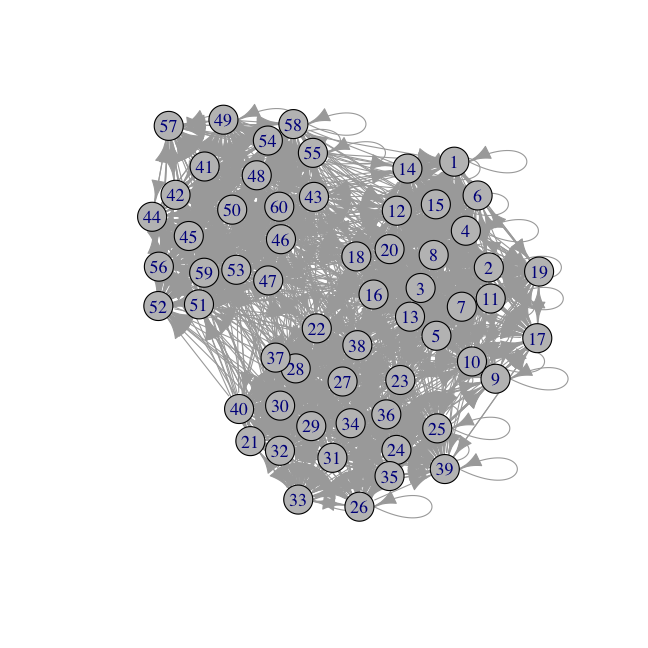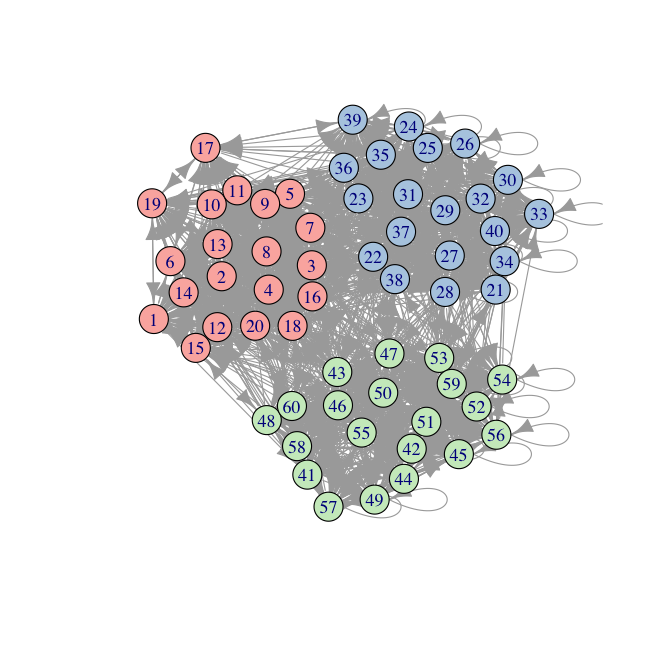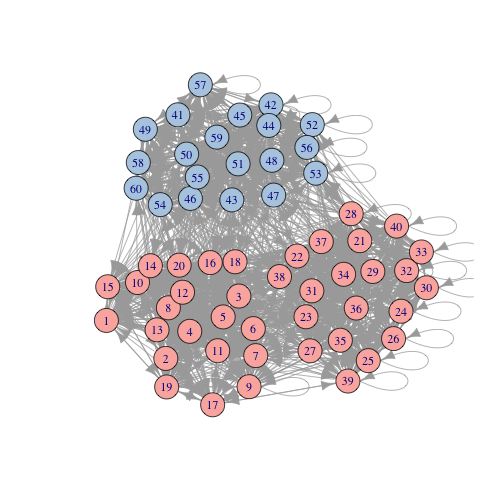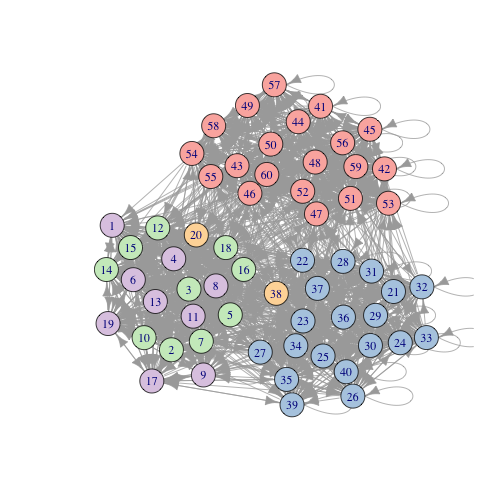## Clustering with the Leiden Algorithm in R

This package allows calling the Leiden algorithm for clustering on an igraph object from R. See the Python and Java implementations for more details:

https://github.com/CWTSLeiden/networkanalysis

https://github.com/vtraag/leidenalg

## Install

This package requires the 'leidenalg' and 'igraph' modules for python (2) to be installed on your system. For example:

`pip install leidenalg igraph`

If you do not have root access, you can use `pip install --user` or `pip install --prefix` to install these in your user directory (which you have write permissions for) and ensure that this directory is in your PATH so that Python can find it.

The 'devtools' package will be used to install 'leiden' and the dependancies (igraph and reticulate). To install the development version:

``````if (!requireNamespace("devtools"))
install.packages("devtools")
devtools::install_github("TomKellyGenetics/leiden")
``````

The current release on CRAN can be installed with:

``````install.packages("leiden")
``````
``````library("leiden")
``````

## Usage

### Running the Leiden algorithm in R

First set up a compatible adjacency matrix:

``````adjacency_matrix <- rbind(cbind(matrix(round(rbinom(400, 1, 0.8)), 20, 20),
matrix(round(rbinom(400, 1, 0.3)), 20, 20),
matrix(round(rbinom(400, 1, 0.1)), 20, 20)),
cbind(matrix(round(rbinom(400, 1, 0.3)), 20, 20),
matrix(round(rbinom(400, 1, 0.8)), 20, 20),
matrix(round(rbinom(400, 1, 0.2)), 20, 20)),
cbind(matrix(round(rbinom(400, 1, 0.3)), 20, 20),
matrix(round(rbinom(400, 1, 0.1)), 20, 20),
matrix(round(rbinom(400, 1, 0.9)), 20, 20)))
str(adjacency_matrix)
#>  num [1:60, 1:60] 1 1 1 1 1 0 0 1 0 1 ...
dim(adjacency_matrix )
#>  60 60
``````

An adjacency matrix is any binary matrix representing links between nodes (column and row names). It is a directed graph if the adjacency matrix is not symmetric.

``````library("igraph")
rownames(adjacency_matrix) <- 1:60
colnames(adjacency_matrix) <- 1:60
graph_object <- graph_from_adjacency_matrix(adjacency_matrix, mode = "directed")
graph_object
#> IGRAPH eace059 DN-- 60 1512 --
#> + attr: name (v/c)
#> + edges from eace059 (vertex names):
#>    1->1  1->3  1->4  1->5  1->6  1->7  1->8  1->9  1->11 1->12 1->13 1->14 1->15 1->16 1->17 1->18 1->19 1->20 1->22
#>   1->27 1->29 1->36 1->38 1->41 1->50 2->1  2->2  2->4  2->5  2->6  2->7  2->8  2->10 2->12 2->13 2->14 2->15 2->16
#>   2->17 2->18 2->20 2->21 2->23 2->24 2->25 2->31 2->49 2->52 2->60 3->1  3->2  3->3  3->4  3->6  3->7  3->9  3->10
#>   3->11 3->12 3->13 3->14 3->15 3->16 3->18 3->19 3->20 3->21 3->26 3->28 3->30 3->33 3->35 3->36 3->37 3->42 3->46
#>   3->49 4->1  4->2  4->3  4->4  4->5  4->6  4->7  4->8  4->9  4->11 4->12 4->13 4->14 4->15 4->16 4->17 4->18 4->19
#>   4->23 4->25 4->26 4->30 4->33 4->43 4->54 4->58 5->1  5->2  5->3  5->4  5->5  5->6  5->7  5->8  5->9  5->10 5->11
#>  5->12 5->13 5->14 5->15 5->16 5->19 5->20 5->23 5->25 5->26 5->29 5->30 5->31 5->34 5->36 5->42 5->44 5->46 6->2
#>  6->3  6->4  6->7  6->8  6->11 6->13 6->14 6->16 6->18 6->19 6->20 6->23 6->28 6->30 6->32 6->40 6->43 7->2  7->3
#> + ... omitted several edges
``````

This represents the following graph structure.

``````plot(graph_object, vertex.color = "grey75")
``````Then the Leiden algorithm can be run on the igraph object.

``````partition <- leiden(graph_object)
``````
``````table(partition)
#> partition
#>  1  2  3
#> 20 20 20
``````

Here we can see partitions in the plotted results. The nodes that are more interconnected have been partitioned into separate clusters.

``````library("RColorBrewer")
node.cols <- brewer.pal(max(c(3, partition)),"Pastel1")[partition]
plot(graph_object, vertex.color = node.cols)
``````### Running Leiden with arguments passed to leidenalg

Arguments can be passed to the leidenalg implementation in Python:

``````#run with defaults
partition <- leiden(graph_object)

#run with ModularityVertexPartition"
partition <- leiden(graph_object, partition_type = "ModularityVertexPartition")

#run with resolution parameter
partition <- leiden(graph_object, resolution_parameter = 0.95)
``````

In particular, the resolution parameter can tune the number of clusters to be detected.

``````partition <- leiden(graph_object, resolution_parameter = 0.5)
node.cols <- brewer.pal(max(c(3, partition)),"Pastel1")[partition]
plot(graph_object, vertex.color = node.cols)
````````````partition <- leiden(graph_object, resolution_parameter = 1.8)
node.cols <- brewer.pal(max(c(3, partition)),"Pastel1")[partition]
plot(graph_object, vertex.color = node.cols)
``````Weights for edges an also be passed to the leiden algorithm either as a separate vector or as a weighted graph_object. Weights will be derived from a weighted graph object.

``````# generate (unweighted) igraph object in R
library("igraph")
adjacency_matrix[adjacency_matrix > 1] <- 1
snn_graph <- graph_from_adjacency_matrix(adjacency_matrix)
partition <- leiden(snn_graph)
table(partition)
#> partition
#>  1  2  3
#> 20 20 20
``````
``````# pass weights to python leidenalg
adjacency_matrix[adjacency_matrix >= 1 ] <- 1
snn_graph <- graph_from_adjacency_matrix(adjacency_matrix, weighted = NULL)
weights <- sample(1:10, sum(adjacency_matrix!=0), replace=TRUE)
partition <- leiden(snn_graph, weights = weights)
table(partition)
#> partition
#>  1  2  3
#> 20 20 20
``````
``````# generate (weighted) igraph object in R
library("igraph")
adjacency_matrix[adjacency_matrix >= 1] <- weights
snn_graph <- graph_from_adjacency_matrix(adjacency_matrix, weighted = TRUE)
partition <- leiden(snn_graph)
table(partition)
#> partition
#>  1  2  3
#> 20 20 20
``````

See the documentation on the leidenalg Python module for more information: https://leidenalg.readthedocs.io/en/latest/reference.html

## Running on a Seurat Object

### Seurat version 2

To use Leiden with the Seurat pipeline for a Seurat Object `object` that has an SNN computed (for example with `Seurat::FindClusters` with `save.SNN = TRUE`). This will compute the Leiden clusters and add them to the Seurat Object Class. The R implementation of Leiden can be run directly on the snn igraph object in Seurat.

``````library("Seurat")
FindClusters(pbmc_small)
membership <- leiden(pbmc_small@snn)
table(membership)
pbmc_small@ident <- as.factor(membership)
names(pbmc_small@ident) <- rownames(pbmc_small@meta.data)
pbmc_small@meta.data\$ident <- as.factor(membership)
``````

Note that this code is designed for Seurat version 2 releases.

### Seurat version 3 or later

Note that the object for Seurat version 3 has changed. For example an SNN can be generated:

``````library("Seurat")
FindClusters(pbmc_small)
membership <- leiden(pbmc_small@graphs\$RNA_snn)
table(membership)
``````

For Seurat version 3 objects, the Leiden algorithm has been implemented in the Seurat version 3 package with `Seurat::FindClusters` and `algorithm = "leiden"`). See the documentation for these functions.

``````FindClusters(pbmc_small, algorithm = "leiden")
table(pbmc_small@active.ident)
``````